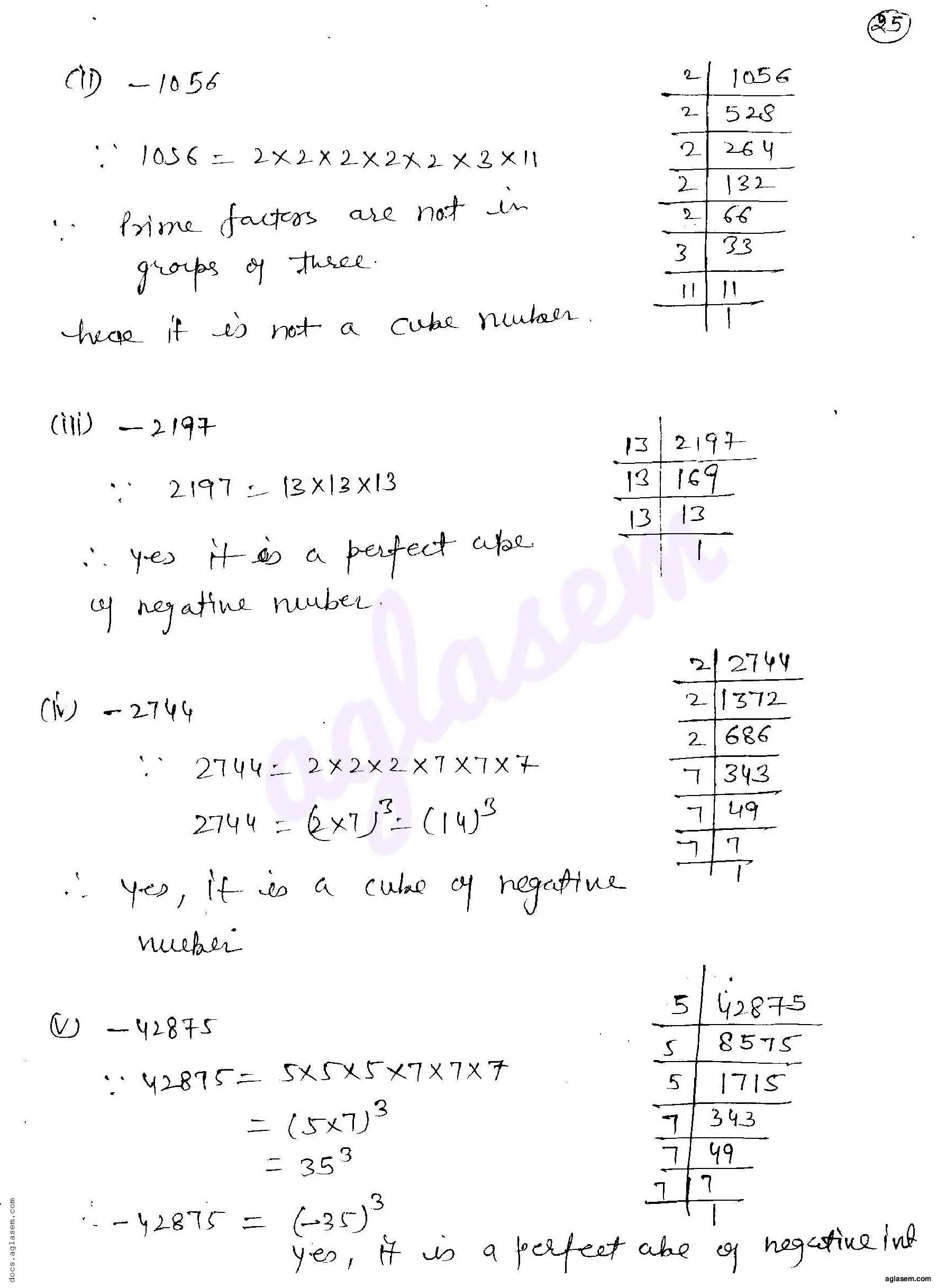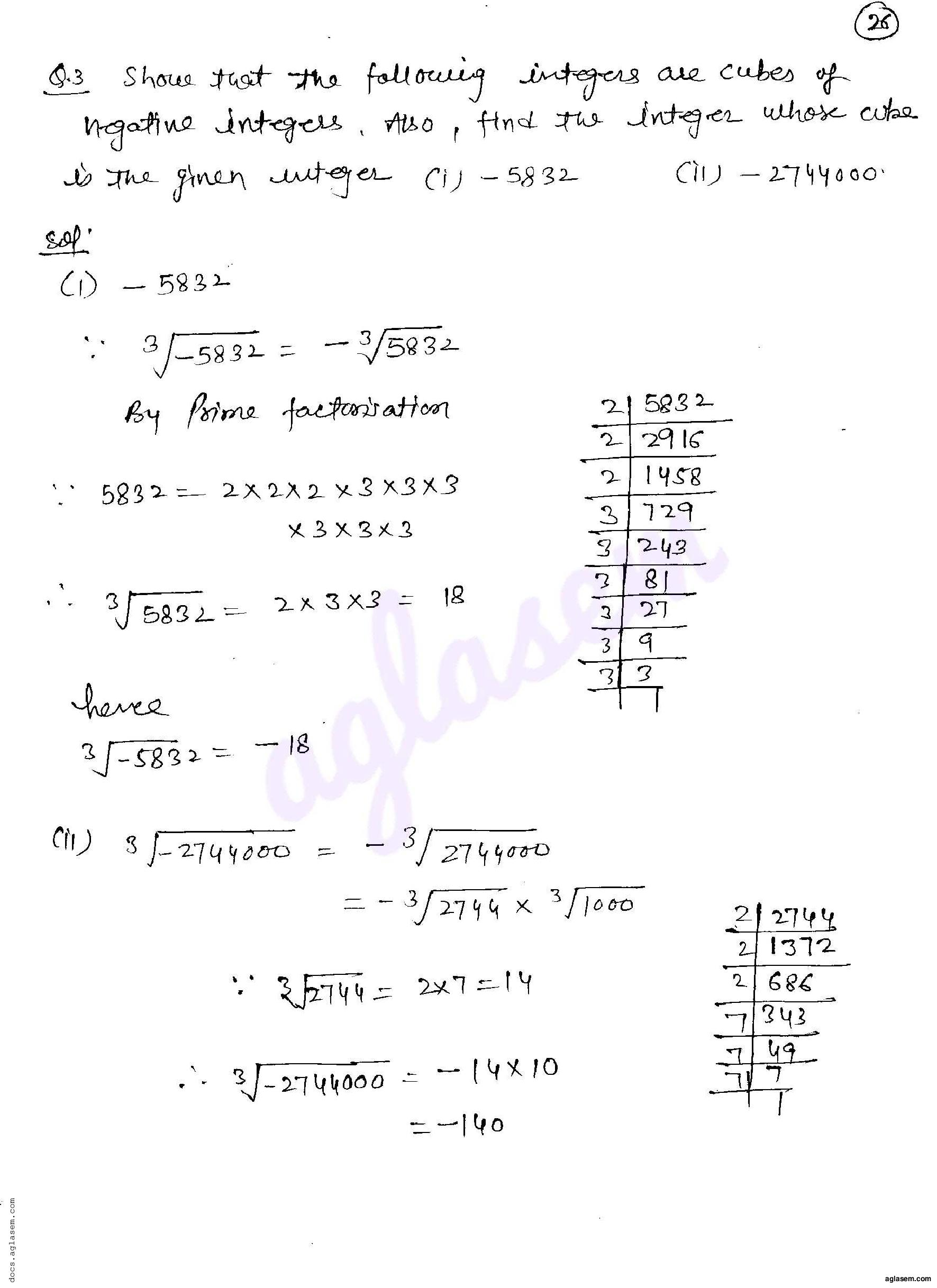# RD Sharma Solutions Class 8 Chapter 4 Cubes and Cube Roots Exercise 4.2

Here you can get free RD Sharma Solutions for Class 8 Maths Chapter 4 Cubes and Cube Roots Exercise 4.2. All RD Sharma Book Solutions are given here exercise wise for the chapter Cubes and Cube Roots. RD Sharma Solutions are helpful in the preparation of several school level, graduate and undergraduate level competitive exams. Practicing questions from RD Sharma Mathematics Solutions for Class 8 Chapter 4 Cubes and Cube Roots is proven to enhance your math skills.

 Class: Class 8th Chapter: Chapter 4 Exercise: Exercise 4.2 Name: Cubes and Cube Roots

## RD Sharma Solutions Class 8 Chapter 4 Cubes and Cube Roots Exercise 4.2

RD Sharma Class 8 Solutions Chapter 4 for Cubes and Cube Roots Exercise 4.2 are given below.

RD Sharma Solutions Class 8 Chapter 4 Cubes and Cube Roots Exercise 4.2RD Sharma Solutions for Class 8 Maths PDF download for Chapter 4 Cubes and Cube Roots Exercise 4.2 is available here for free.

 « Previous Next »

### RD Sharma Solutions Class 8 Chapter 4 Exercise Wise

You can also see RD Sharma Solutions of all exercises of Chapter 4 – Cubes and Cube Roots from here.

### RD Sharma Solutions Class 8 Chapter Wise

If you want RD Sharma solutions of any topic other than Chapter 4 Cubes and Cube Roots, check it from here. Mathematics by RD Sharma has all chapters that are in your Class 8 syllabus.

Click to rate this post!
[Total: 2 Average: 5]